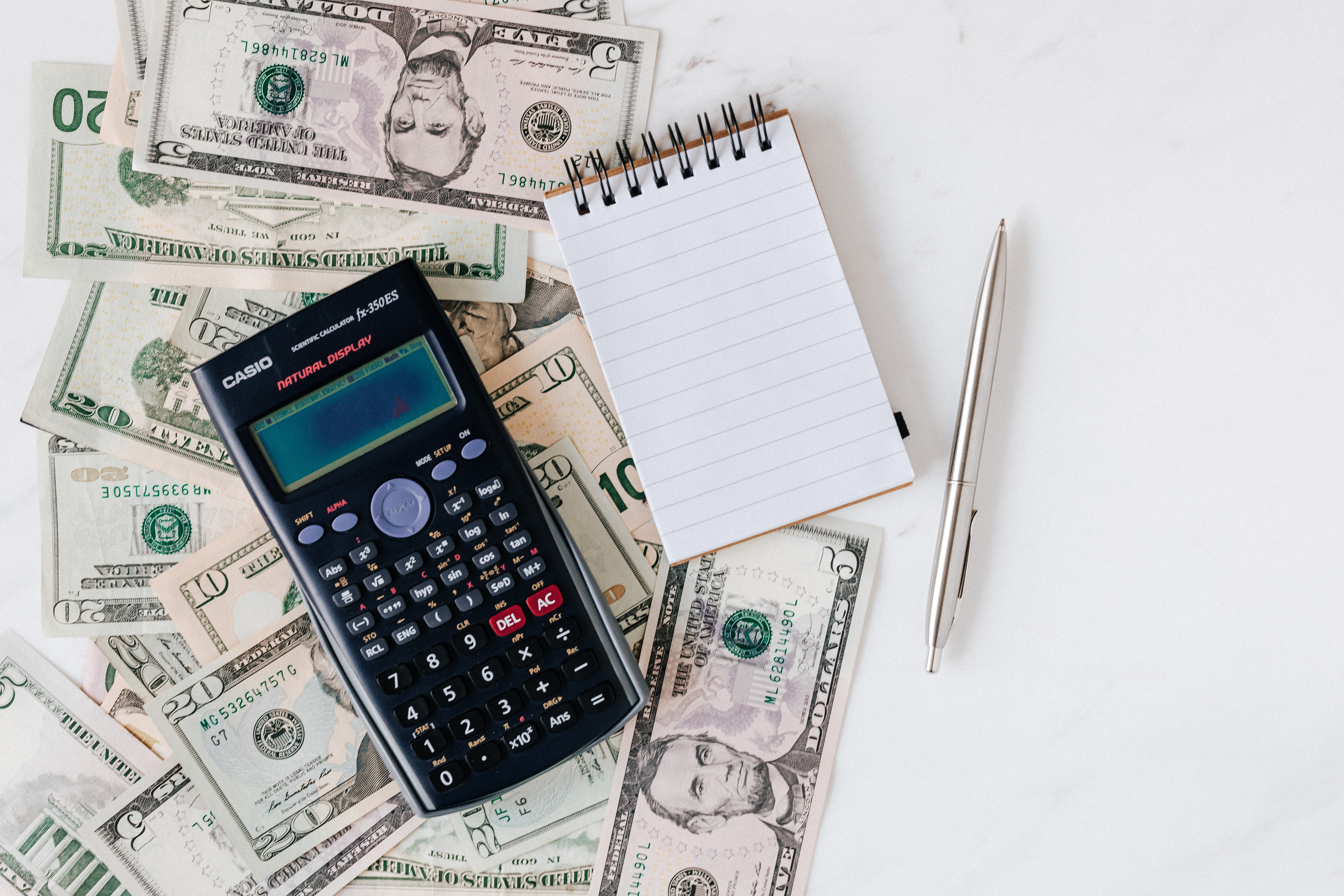# How are airline miles worth calculated?

To calculate the value of an airline mile, points brokers and travel websites use their own personal mathematical formulas. The value of a mile is approximate and constantly changing. There is a lot of confusion about how airline miles worth is calculated!

Many people choose to sell miles for cash because of decreasing points values.

However, you can calculate the worth of your miles and see if it’s still worth collecting them.

## There is no specific formula

Different evaluators use different calculation methods because there is no universal scientific or mathematical formula one can use. Each person can use his or her preferred method, but as you’ll see below, the numbers are fairly similar.

The best method to calculate the value of airline miles is to determine the value for a specific ticket and use a mathematical formula to give it a monetary value, usually in cents. Most airline miles are valued between 1-2 cents USD.

Note that determining the value of an airline mile requires generalization. There is no specific or universally accepted method. The values are based on average rates.

There is one way that most people calculate the value of an airline point or mile.

### Price of a specific flight divided by the price of a ticket in miles

Divide the price of a specific flight (in USD) by the price of the ticket in frequent flyer miles (USD).

For example, a flight that costs \$7000 divided by the cost in frequent flyer miles of 70,000 = 0.10.

But you might notice that airline miles are usually valued somewhere between 0.50 – 2 cents per point.

The reason is that there is a secondary but more complex formula to calculate airline points values.

When you divide the price for each flight by the number of miles it takes to travel that specific route, you’ll notice a common trend: there is a disparity between the value of miles for domestic versus international flights.

For example, an American Airlines AAdvantage mile is worth approximately 1 cent USD for domestic flights and approximately 1.8 cents USD for international flights.

## Determining the value of a point: consider other factors

Compare how much cash it takes to buy points, the value of redeeming them, and then factor in variables like cancellation policies, extra fees, and availability. Also, with the coronavirus pandemic, many airlines and hotels are offering major discounts and the chance to buy points at discounted rates. This is the result of diminished travel and dropping point values for major airlines.

The bottom line is that while there’s no specific formula to calculate airline miles, websites and points brokers use similar mathematical formulas to determine value based on a variety of factors.